# Chapter 1 Matrices Definition 1 A matrix is

• Slides: 12
Download presentationChapter 1: Matrices Definition 1: A matrix is a rectangular array of numbers arranged in horizontal rows and vertical columns. EXAMPLE: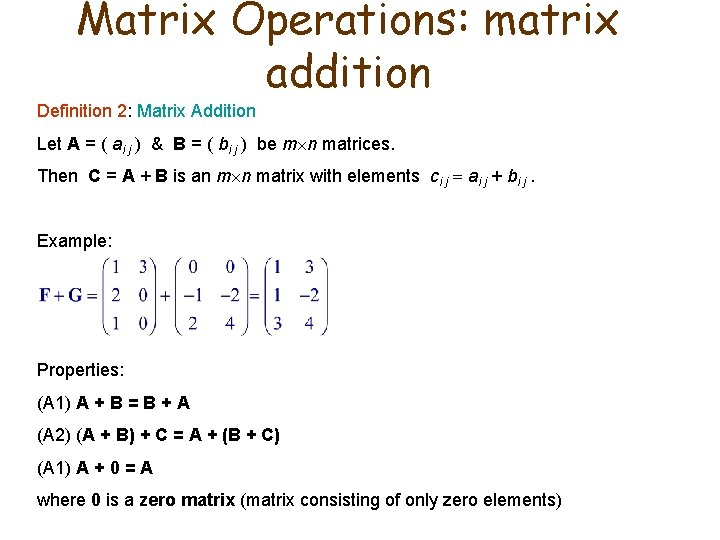Matrix Operations: matrix addition Definition 2: Matrix Addition Let A = ( ai j ) & B = ( bi j ) be m n matrices. Then C = A + B is an m n matrix with elements ci j ai j + bi j. Example: Properties: (A 1) A + B = B + A (A 2) (A + B) + C = A + (B + C) (A 1) A + 0 = A where 0 is a zero matrix (matrix consisting of only zero elements)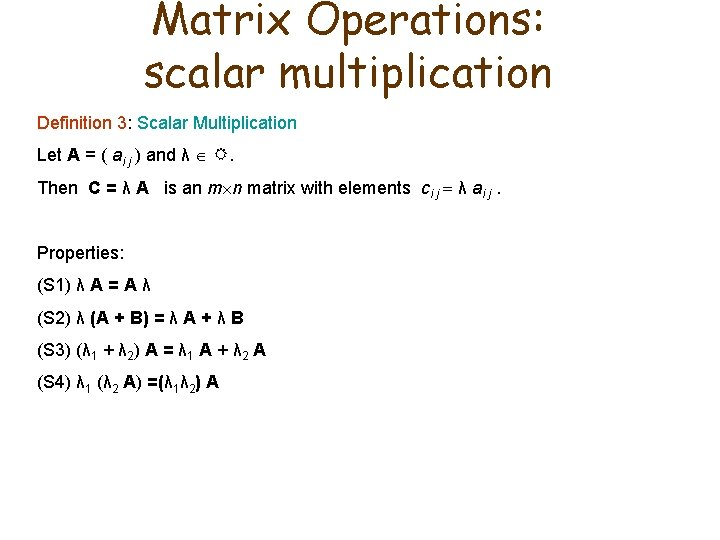Matrix Operations: scalar multiplication Definition 3: Scalar Multiplication Let A = ( ai j ) and λ R. Then C = λ A is an m n matrix with elements ci j λ ai j. Properties: (S 1) λ A = A λ (S 2) λ (A + B) = λ A + λ B (S 3) (λ 1 + λ 2) A = λ 1 A + λ 2 A (S 4) λ 1 (λ 2 A) =(λ 1λ 2) A1. 3 Matrix Multiplication Definition: Matrix Multiplication If A = ( ai j ) is an m n matrix and B = ( bi j ) is an n p matrix, Then the product AB is defined to be an m p matrix C = ( ci j ) where ci j = ai 1 b 1 j + ai 2 b 2 j + … + ai n bn j In other words, ci j = ( Row i of A ) ( Column j of B ) Example: Properties: (M 1) (AB)C = A(BC) (M 2) A(B + C) = AB + AC (M 3) (B + C)A = BA + CAMatrix multiplication lacks commutativity. Example 1: Example 2: Other properties that matrix multiplication lacks: 1) AB = 0 doesn’t imply A = 0 or B = 0 2) AB = AC doesn’t imply B = C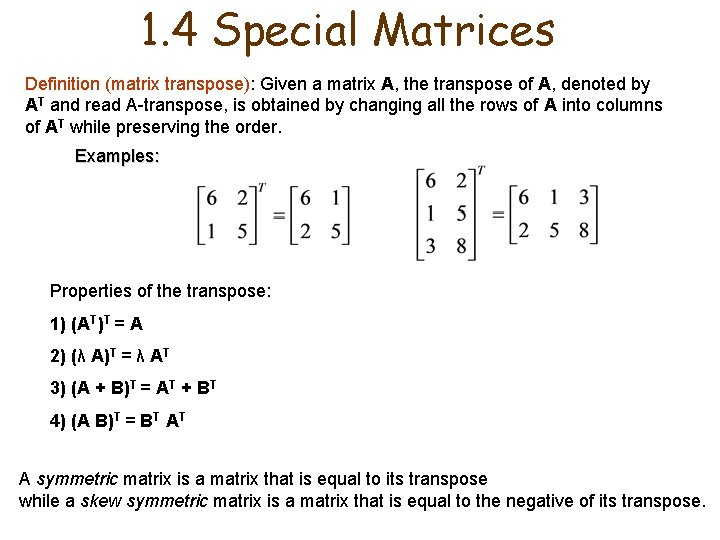1. 4 Special Matrices Definition (matrix transpose): Given a matrix A, the transpose of A, denoted by AT and read A-transpose, is obtained by changing all the rows of A into columns of AT while preserving the order. Examples: Properties of the transpose: 1) (AT)T = A 2) (λ A)T = λ AT 3) (A + B)T = AT + BT 4) (A B)T = BT AT A symmetric matrix is a matrix that is equal to its transpose while a skew symmetric matrix is a matrix that is equal to the negative of its transpose.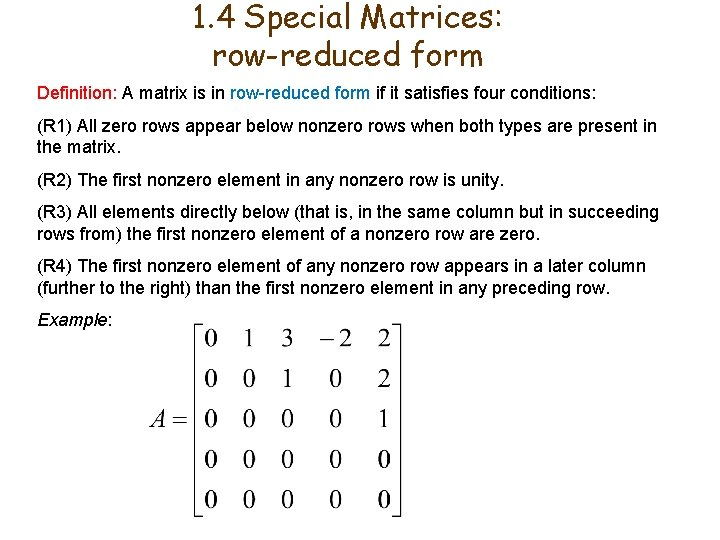1. 4 Special Matrices: row-reduced form Definition: A matrix is in row-reduced form if it satisfies four conditions: (R 1) All zero rows appear below nonzero rows when both types are present in the matrix. (R 2) The first nonzero element in any nonzero row is unity. (R 3) All elements directly below (that is, in the same column but in succeeding rows from) the first nonzero element of a nonzero row are zero. (R 4) The first nonzero element of any nonzero row appears in a later column (further to the right) than the first nonzero element in any preceding row. Example: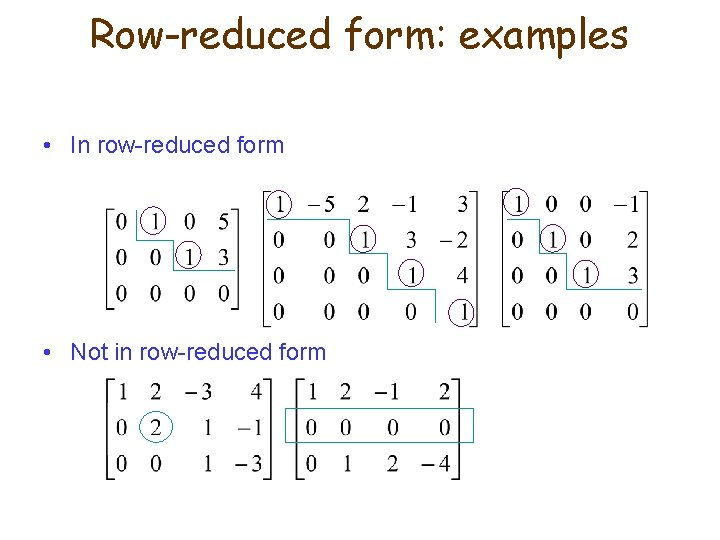Row-reduced form: examples • In row-reduced form • Not in row-reduced form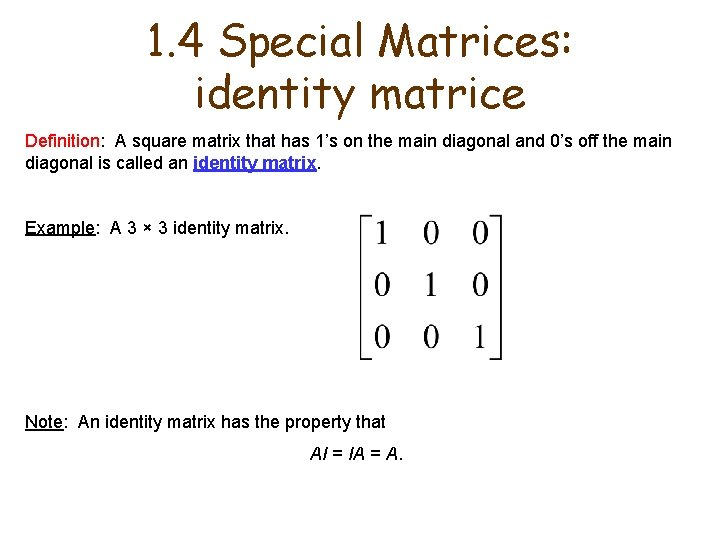1. 4 Special Matrices: identity matrice Definition: A square matrix that has 1’s on the main diagonal and 0’s off the main diagonal is called an identity matrix. Example: A 3 × 3 identity matrix. Note: An identity matrix has the property that AI = IA = A.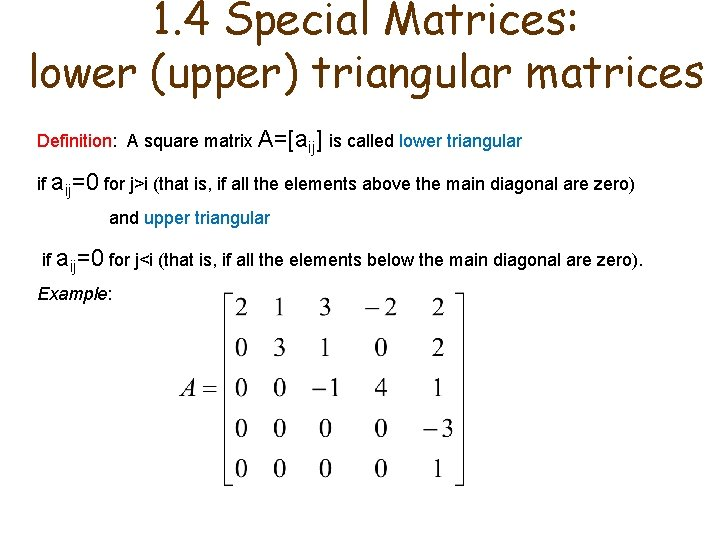1. 4 Special Matrices: lower (upper) triangular matrices Definition: A square matrix A=[aij] is called lower triangular if aij=0 for j>i (that is, if all the elements above the main diagonal are zero) and upper triangular if aij=0 for j<i (that is, if all the elements below the main diagonal are zero). Example: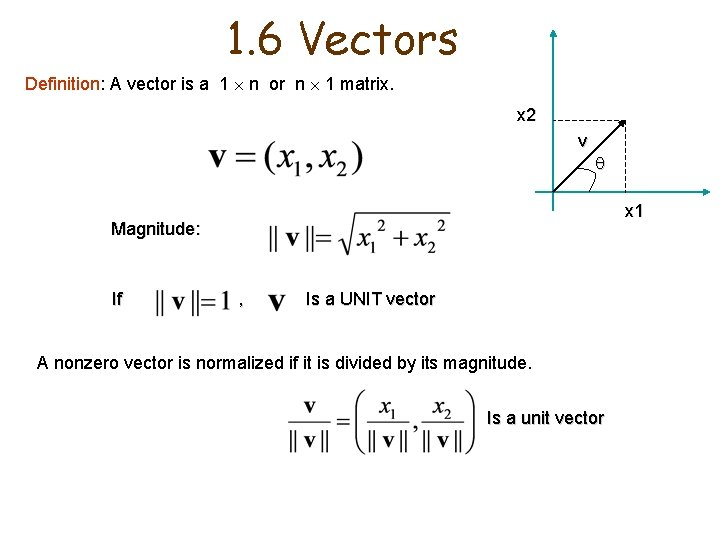1. 6 Vectors Definition: A vector is a 1 n or n 1 matrix. x 2 v x 1 Magnitude: If , Is a UNIT vector A nonzero vector is normalized if it is divided by its magnitude. Is a unit vector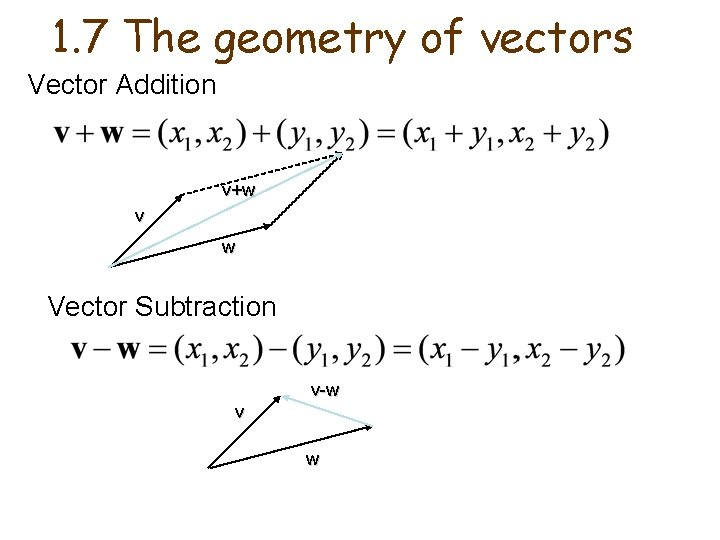1. 7 The geometry of vectors Vector Addition v+w v w Vector Subtraction v-w v w